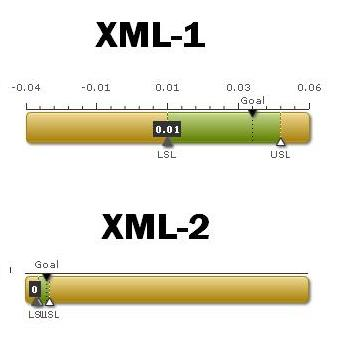# Linear Gauge limits are not proper when pointer value is 0 and limits are decimals

## Recommended Posts

We are currently using FusionWidgets v3.

I am trying to use HLinearGauge.swf to create Linear Gauge on my web page. I am setting lowerLimit of chart to -0.04 and upperLimit to 0.06. I have a pointer to be shown on the chart.

If I set my pointer value to 0.01, chart is rendered properly. If I set it to 0, chart is not rendering properly.

Here are my XML files...

XML-1 Pointer value is set to 0.01 and its working

<Chart bgColor='FFFFFF' bgAlpha='0' valueAbovePointer='1' showBorder='0' upperLimit='0.06' lowerLimit='-0.04' gaugeRoundRadius='5' tickValueDistance='8' trendValueDistance='1' ticksBelowGauge='0' pointerOnTop='0' pointerRadius='5'>

<colorRange>

<color minValue='-0.04' maxValue='0.010' code='d5a615' />

<color minValue='0.010' maxValue='0.050' code='7ca308'  />

<color minValue='0.050' maxValue='0.06' code='d5a615' />

</colorRange>

<pointers>

<pointer value='0.01'/>

</pointers>

<trendpoints>

<point value='0.010' displayValue='LSL' dashed='1' dashLen='1' useMarker='1' markerTooltext='LSL: 0.010' dashGap='3' color='FFFFFF' thickness='1' />

<point value='0.040' showOnTop='1' displayValue='Goal' dashed='1' dashLen='1' useMarker='1' markerTooltext='Goal: 0.040' dashGap='3' color='000000' thickness='1' />

<point value='0.050' displayValue='USL' dashed='1' dashLen='1' useMarker='1' markerTooltext='USL: 0.050' dashGap='3' color='FFFFFF' thickness='1' />

</trendpoints>

<styles>

<definition>

<style name='ValueFont' type='Font' bgColor='333333' size='10' color='FFFFFF' />

</definition>

<application>

<apply toObject='VALUE' styles='valueFont' />

</application>

</styles>

</Chart>

XML- 2 Pointer value is set to 0 and its not working

<Chart bgColor='FFFFFF' bgAlpha='0' valueAbovePointer='1' showBorder='0' upperLimit='0.06' lowerLimit='-0.04' gaugeRoundRadius='5' tickValueDistance='8' trendValueDistance='1' ticksBelowGauge='0' pointerOnTop='0' pointerRadius='5'>

<colorRange>

<color minValue='-0.04' maxValue='0.010' code='d5a615' />

<color minValue='0.010' maxValue='0.050' code='7ca308'  />

<color minValue='0.050' maxValue='0.06' code='d5a615' />

</colorRange>

<pointers>

<pointer value='0'/>

</pointers>

<trendpoints>

<point value='0.010' displayValue='LSL' dashed='1' dashLen='1' useMarker='1' markerTooltext='LSL: 0.010' dashGap='3' color='FFFFFF' thickness='1' />

<point value='0.040' showOnTop='1' displayValue='Goal' dashed='1' dashLen='1' useMarker='1' markerTooltext='Goal: 0.040' dashGap='3' color='000000' thickness='1' />

<point value='0.050' displayValue='USL' dashed='1' dashLen='1' useMarker='1' markerTooltext='USL: 0.050' dashGap='3' color='FFFFFF' thickness='1' />

</trendpoints>

<styles>

<definition>

<style name='ValueFont' type='Font' bgColor='333333' size='10' color='FFFFFF' />

</definition>

<application>

<apply toObject='VALUE' styles='valueFont' />

</application>

</styles>

</Chart>

The only difference in these two xml files is the highlighted pointer value. Try to use these xml files and create a Linear Gauge with HLinearGauge.swf file and see the difference.

Please let me know the work around for this. Thanks.

##### Share on other sites

Here is the output from both the XMLs.